## Outline: Time Series in Practice

• When and why do we need time series models?
• Basic models and definitions: white noise, AR1, MA, random walk, stationarity.
• 3 approaches to time series modelling: ARIMA, Regression, Structural time series / state-space models

understand basic difficulties with time series, construct a few simple but useful models

## Motivating Example: Mauna Loa Atmospheric CO2 Concentration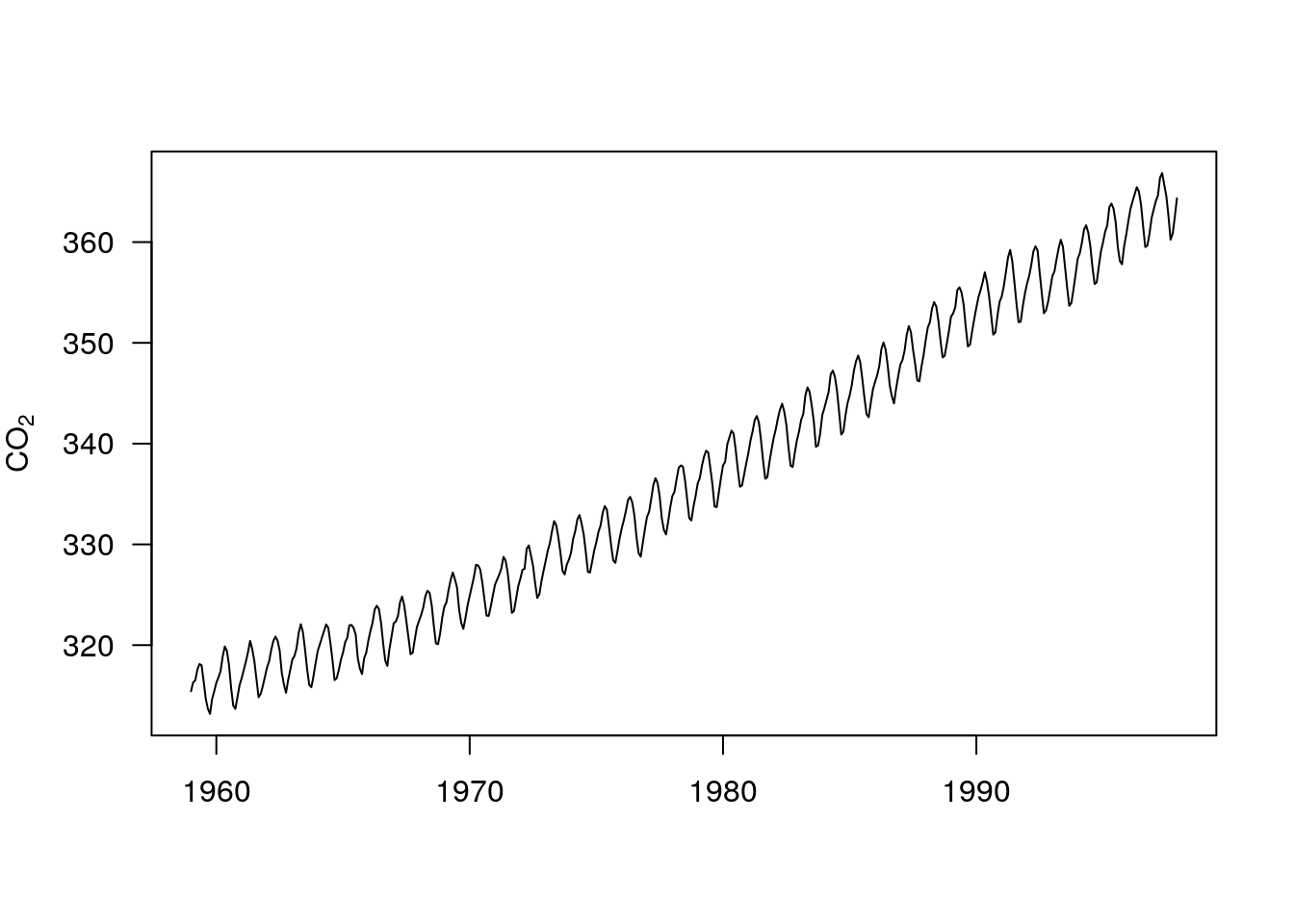## Shark Attacks in Florida

Source: R package bsts (Bayesian structural time series)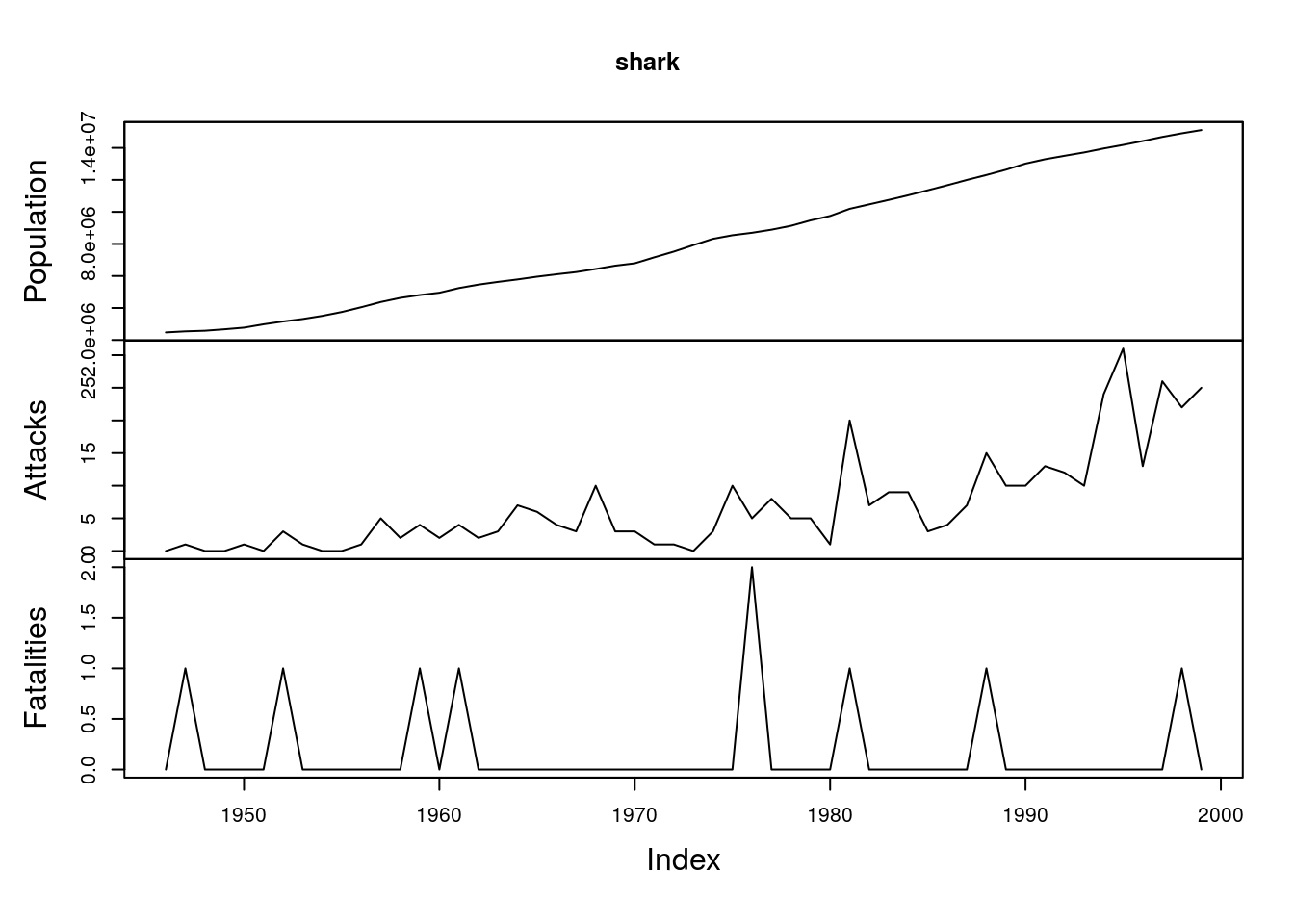## Financial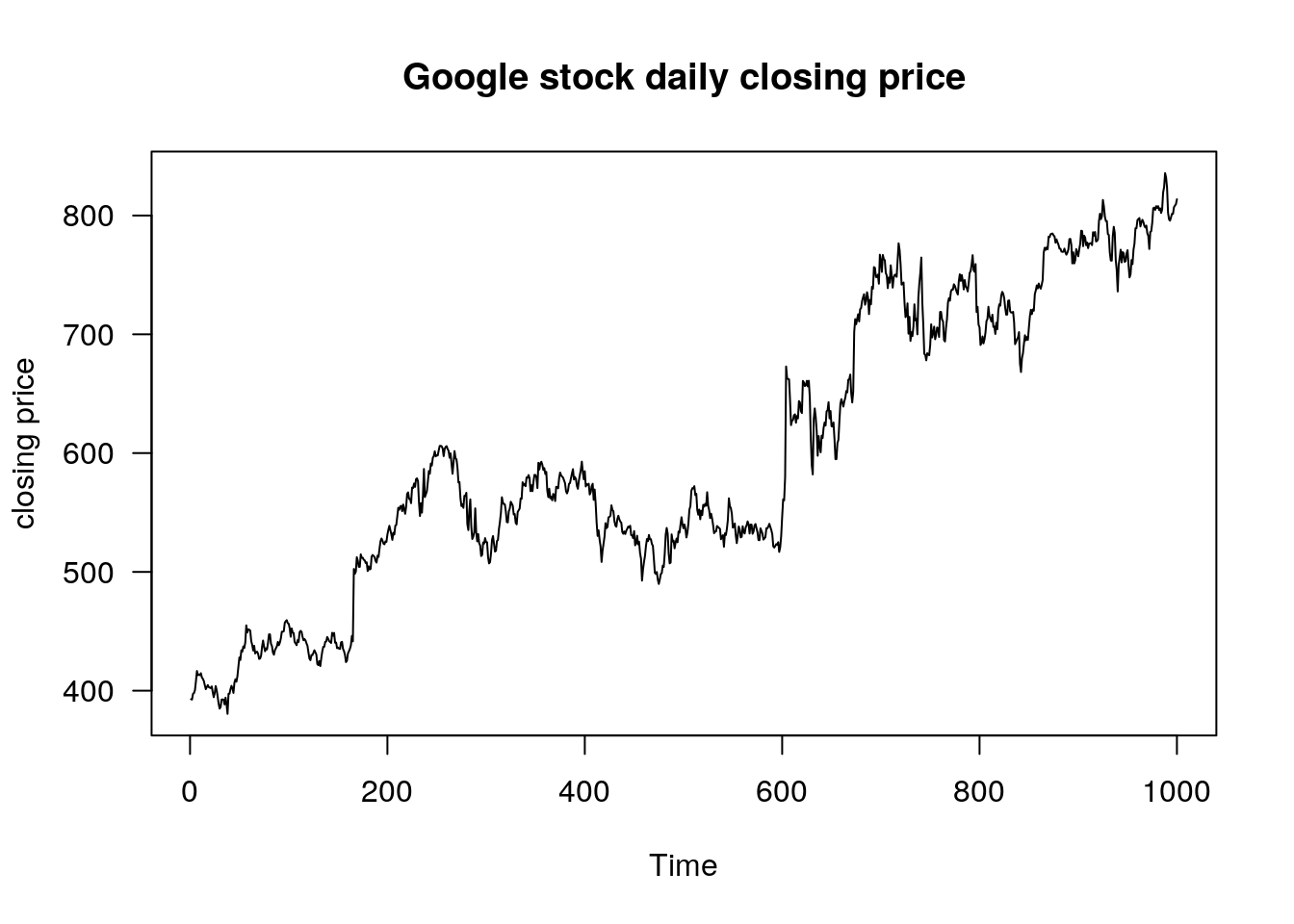fpp2 is the package for the book by Hyndman: Forecasting: Principles and Practice, 2nd edition.

## Sunspot Area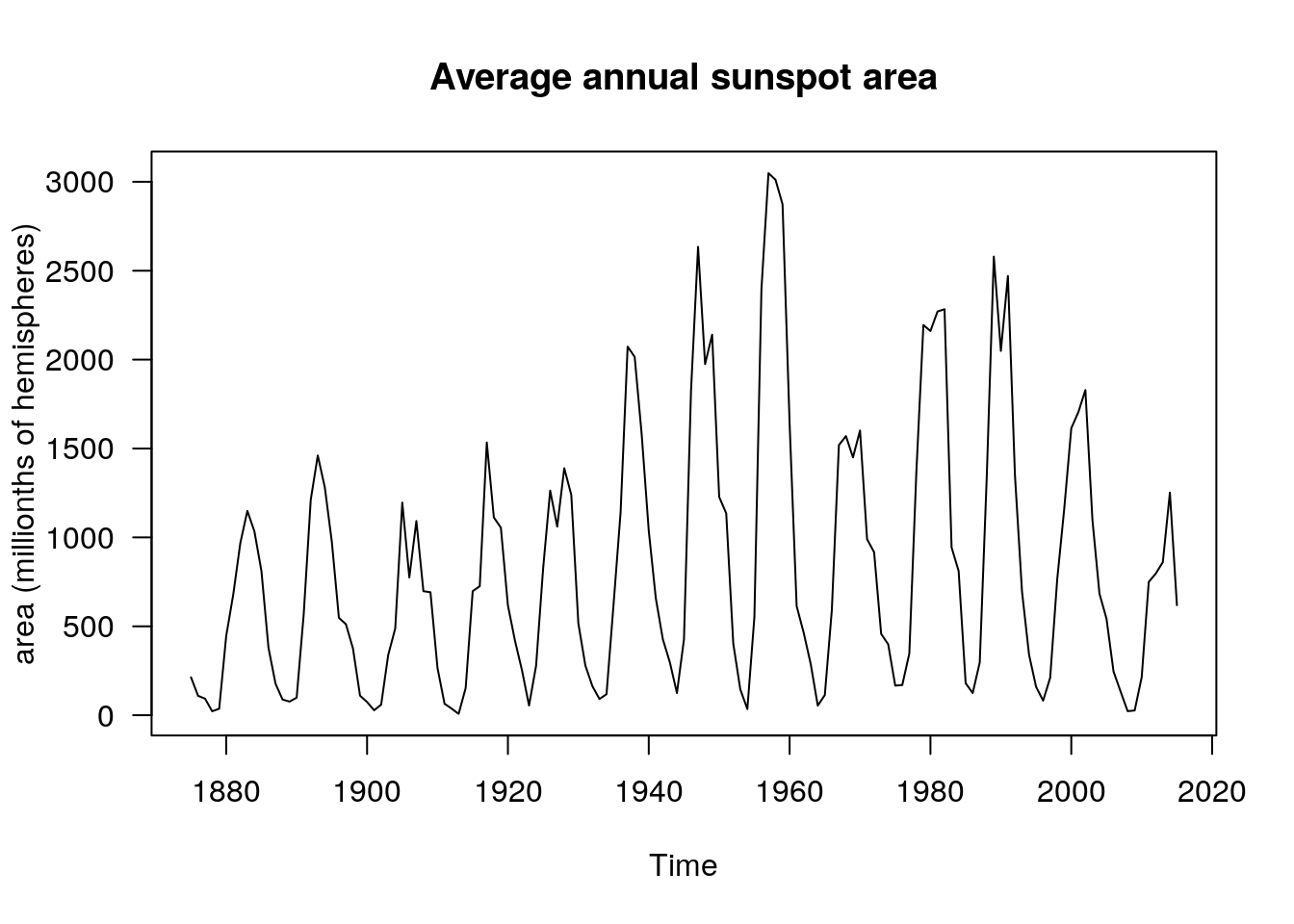## Electricity Production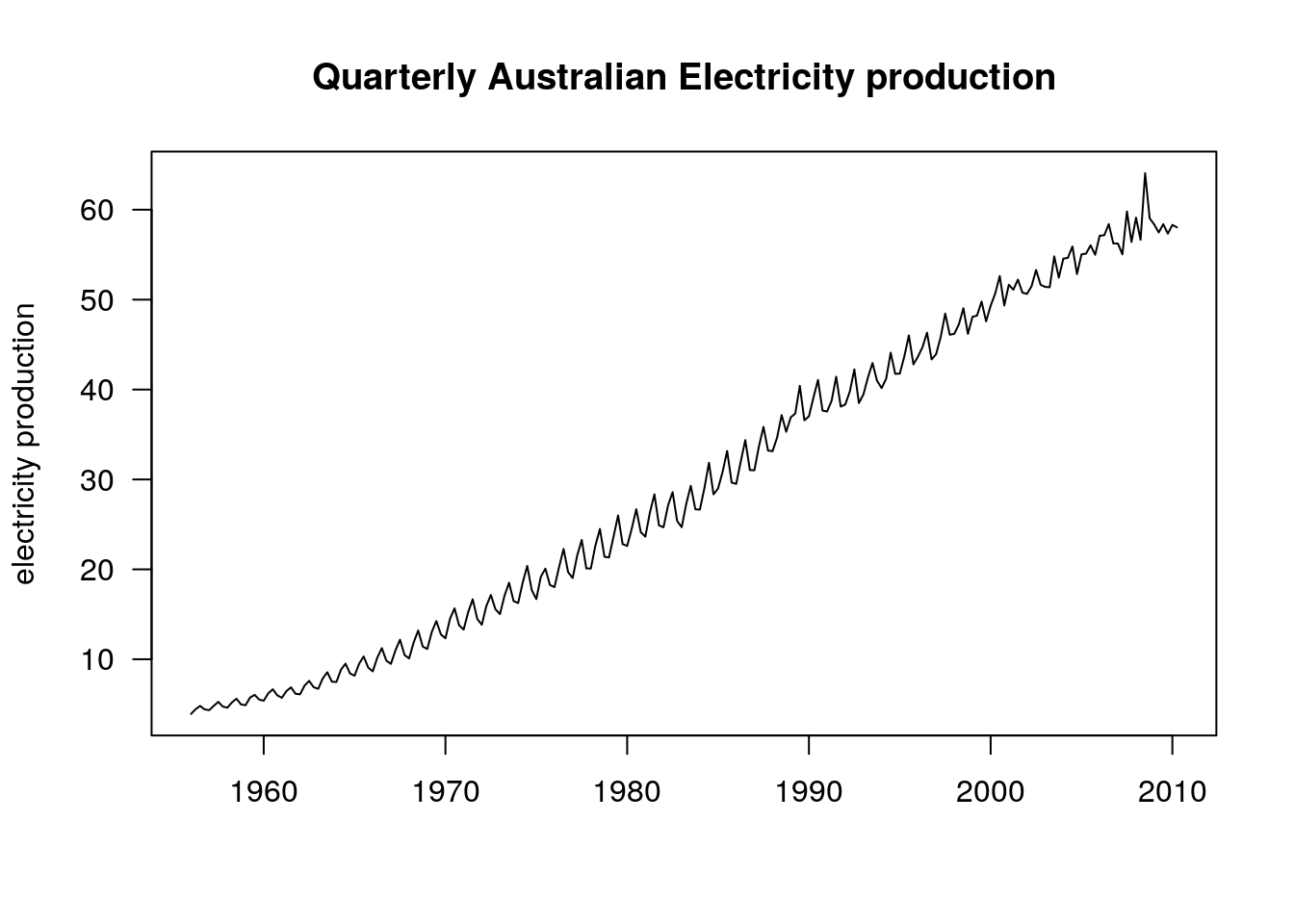## Treerings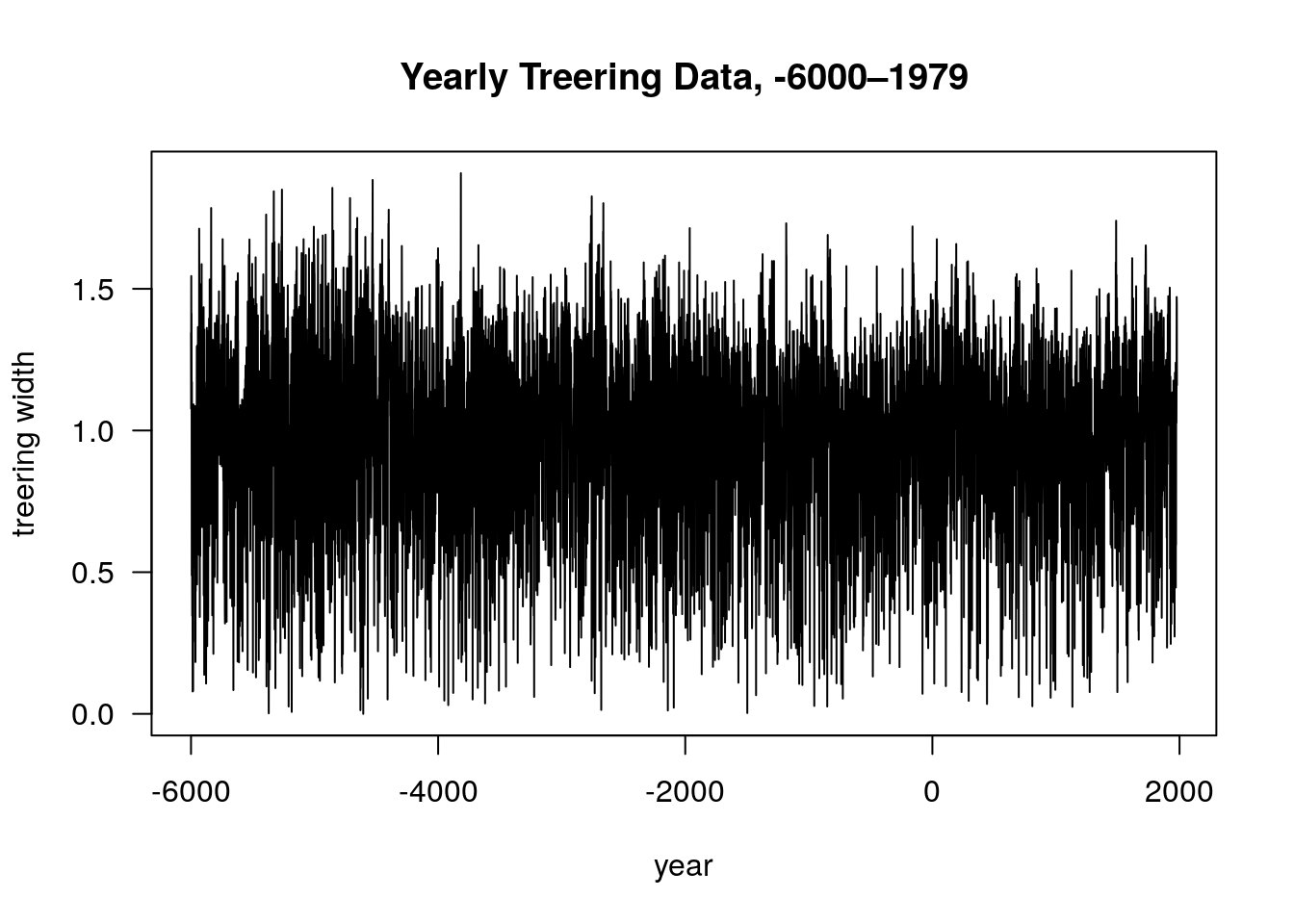## Electricity Demand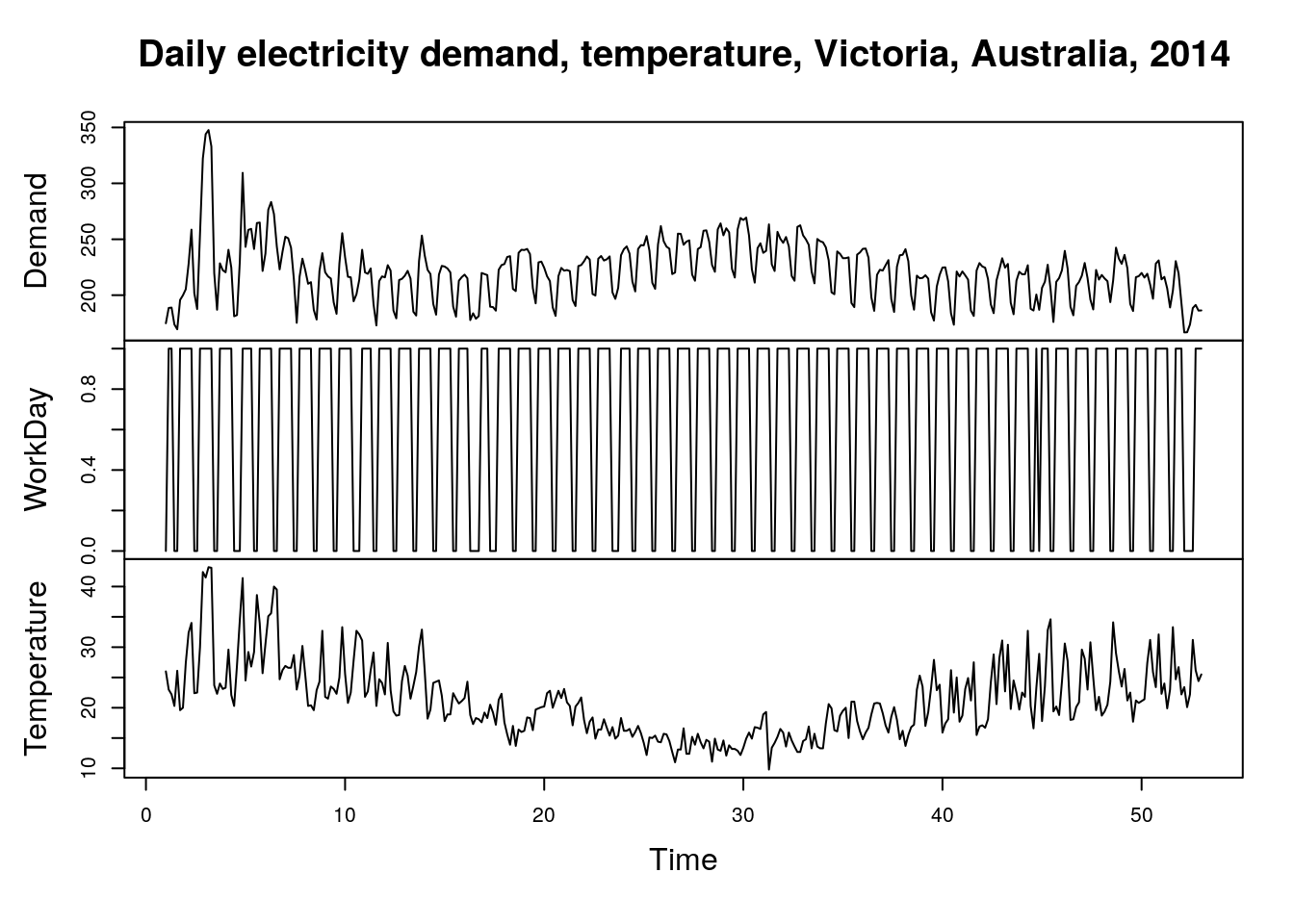## Goals of Time Series Analysis

• prediction / forecast
• impact of single event
• study causal patterns
• detect trends, changes, shifts (in mean, seasonality, variance)

## Time Series in R

Create time series of quarterly data:

ts(rnorm(40), frequency = 4, start = c(2019, 2))

## When and why do we need time series models?

When there is auto-correlation in the residuals (after modelling trends, seasonality, effects of explanatory variables).

If we ignore autocorrelation:

• standard error estimates are wrong

• predictions and prediction intervals are wrong

## Terminology / Definitions

time series: $$y_1, ..., y_t$$

autocorrelation: correlation with previous values,

## Basic time series processes

AR process (auto-regressive)

$\mbox{AR(p):} \qquad x_t = \phi_1 x_{t-1} + \phi_2 x_{t-2} + ... + \phi_p x_{t-p} + e_t$

$\mbox{AR1:} \qquad x_t = \phi x_{t-1} + e_t$

Why would anything behave like this?

I see $$x_{t-1}$$ as a measure of everything that was not measured explicitly at previous time step.

Random walk

$x_t = x_{t-1} + e_t$

## AR1 processes, different $$\phi$$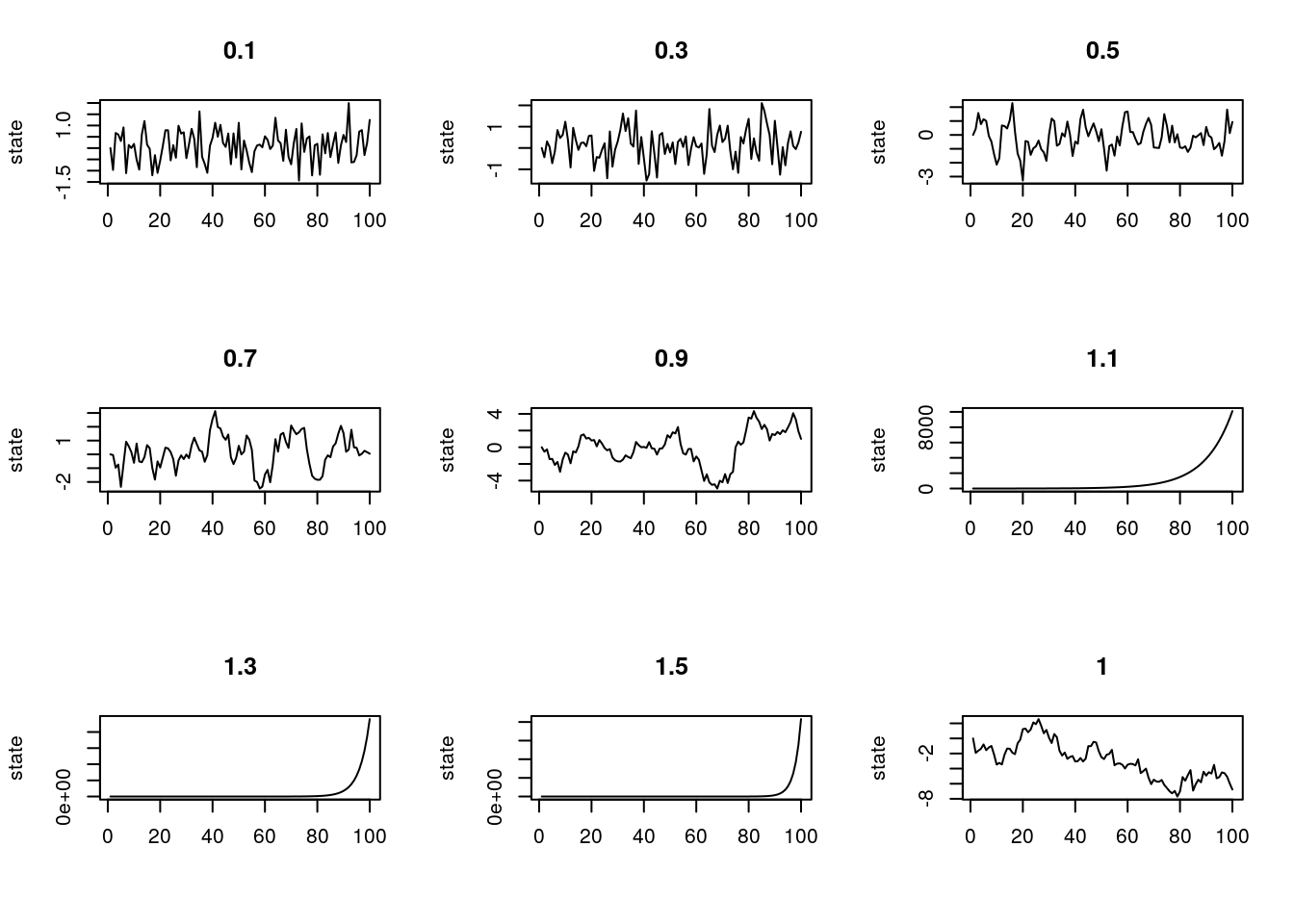## Stationarity

• mean, variance, correlations stay constant over time

Why is stationarity important?

There is a single observation per time point. If mean and variance are different for every point, we can’t estimate mean and variance, correlation or model parameters.

AR1 processes are stationary if $$|\phi| < 1$$.

non-stationarity means

mean changes, variance changes, seasonality present, correlation changes

## MA(q) process (moving average)

$y_t = \theta_1 e_{t-1} + \theta_2 e_{t-2} + \ldots + \theta_p e_{t-p} + e_t$

sum of previous shocks / events

White noise

identically, independently distributed, mean 0, no autocorrelation

## ACF and PACF

autocorrelation function, partial autocorrelation function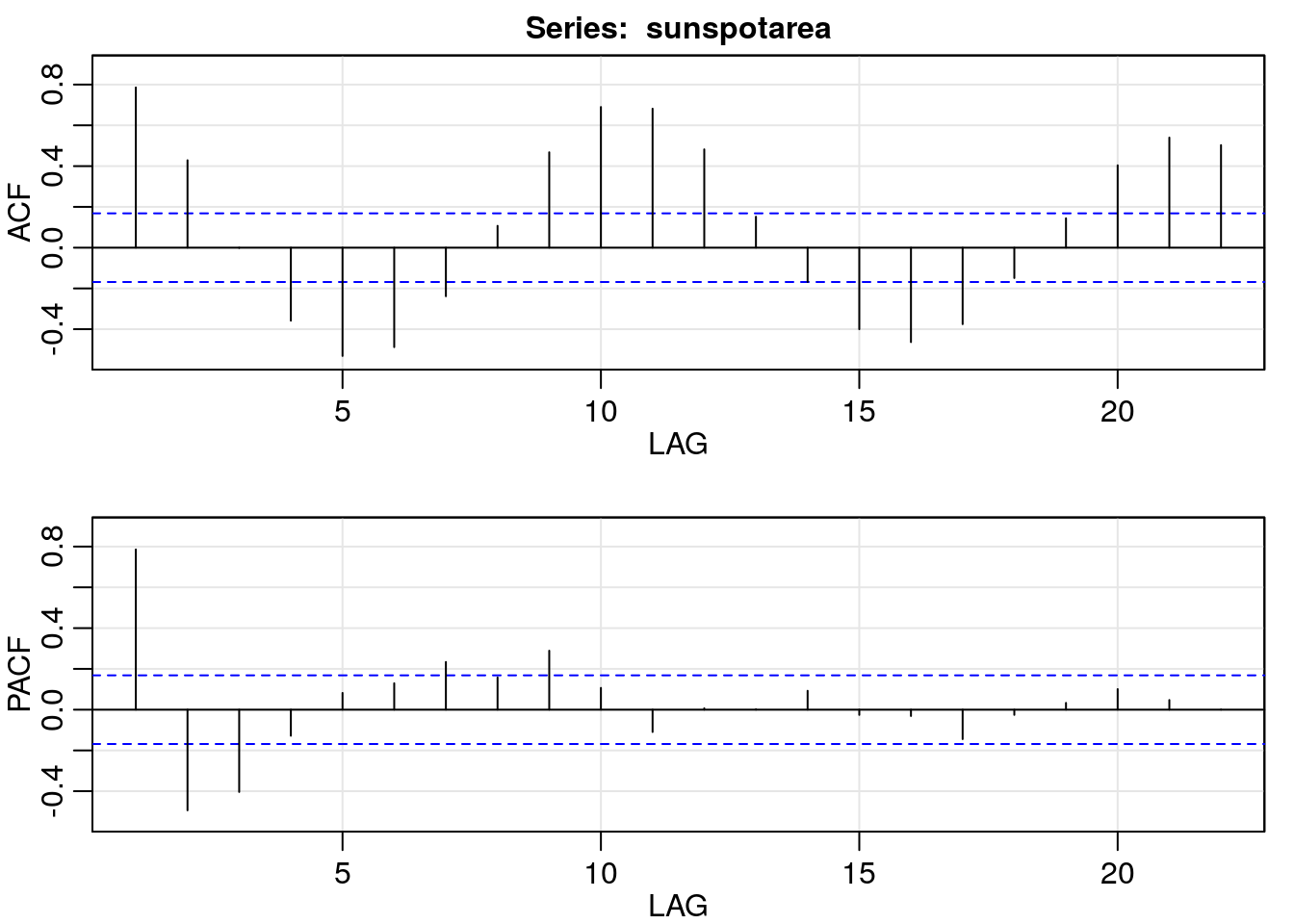partical autocorrelation is the correlation between $$z_t$$ and $$z_{t+k}$$ that is not accounted for by lags 1 to k.

## Three approaches to time series modelling

1. ARIMA, very briefly

arima(p, d, q)

p = order of autoregressive process, d = difference order, q = order of moving average process

If $$y_t$$ is not stationary then $$y_t - y_{t-1}$$ sometimes is (first order differences).

ARIMA: auto-regressive, integrated, moving average

‘integrated’ refers to differencing

## Three approaches to time series modelling

2. Regression

Ignore or model auto-correlation in errors like this:

$y_t = \beta_0 + \beta_1 x_t + \nu_t$

$\nu_t = \phi \nu_{t-1} + e_t$

Not this:

$y_t = \beta_0 + \beta_1 x_t + \phi y_{t-1} + e_t$

problem: $$\beta_1$$ is not change in response per unit change in $$x$$

https://robjhyndman.com/hyndsight/arimax/

## Simulate some data with autocorrelation, fit regression model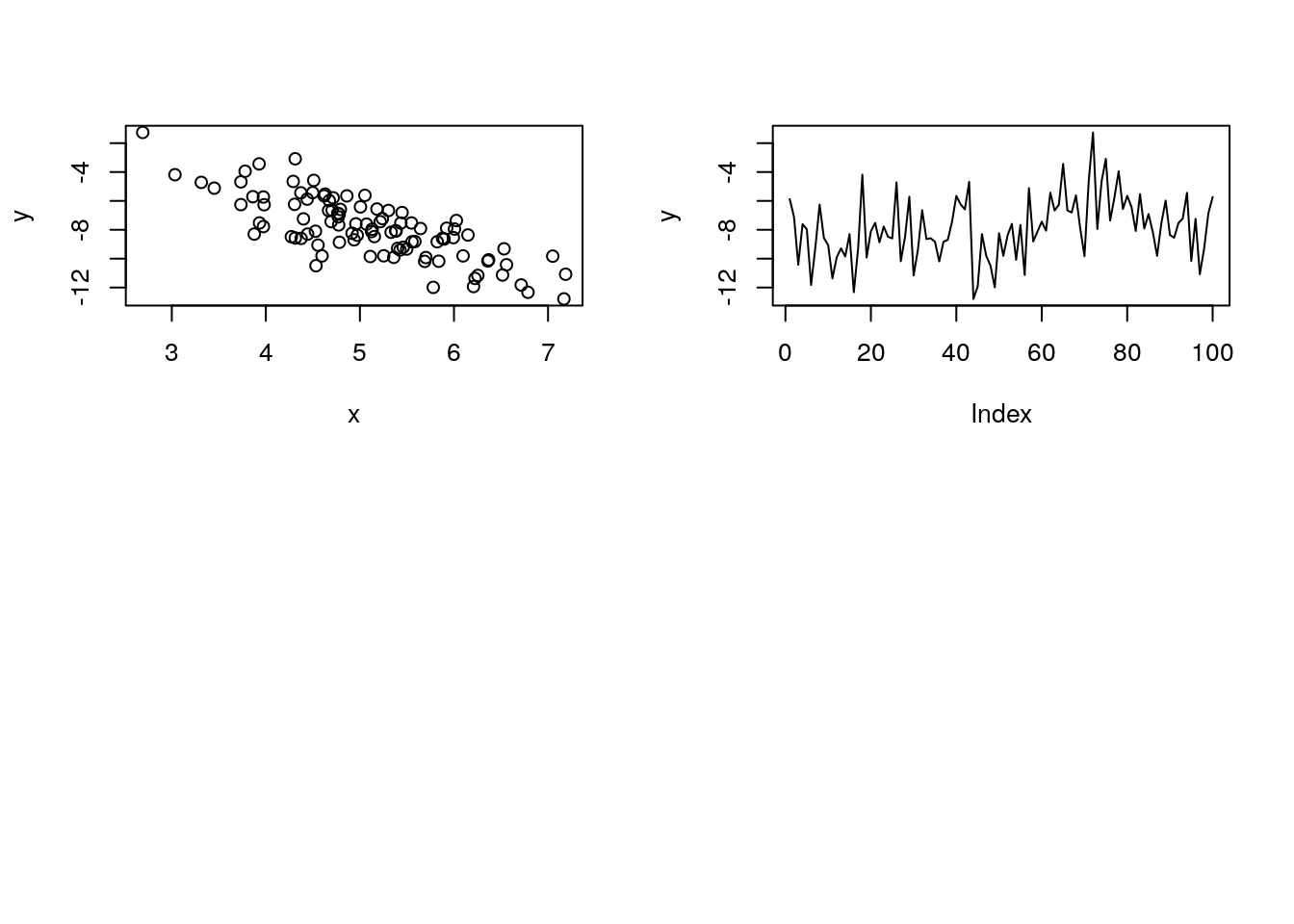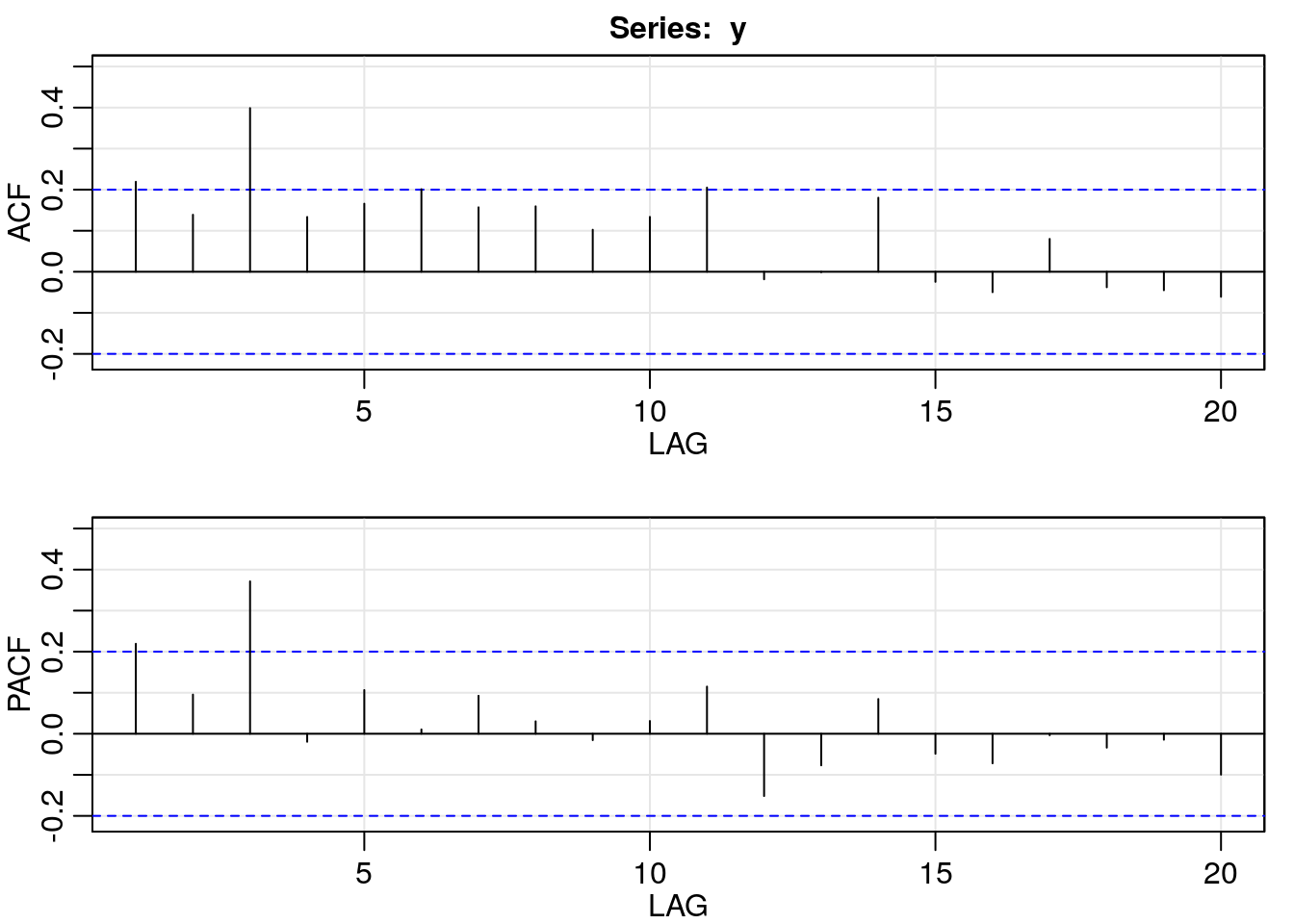acf2comes from package astsa, and skips the auto-correlation at lag 1.

## ARMA errors

arimawith xreg models autocorrelation in errors

a1 <- arima(y, order = c(0, 0, 0), xreg = x)
a2 <- arima(y, order = c(1, 0, 0), xreg = x)
##
## Call:
## arima(x = y, order = c(1, 0, 0), xreg = x)
##
## Coefficients:
##          ar1  intercept        x
##       0.8238     2.1524  -1.9644
## s.e.  0.0544     0.5223   0.0640
##
## sigma^2 estimated as 0.5757:  log likelihood = -114.85,  aic = 237.7

Compare to true values: $$\beta_0 = 3, \beta_1 = -2, \phi = 0.9, \sigma^2 = 0.64$$

## Regression without ARMA errors

##
## Call:
## arima(x = y, order = c(0, 0, 0), xreg = x)
##
## Coefficients:
##       intercept        x
##          1.4424  -1.8305
## s.e.     0.7726   0.1494
##
## sigma^2 estimated as 1.842:  log likelihood = -172.43,  aic = 350.86

Coefficient estimates are further from true values.